# How to Use Countif in VBA in Microsoft Excel

In this article, we will learn how to work on vba and macros in Excel. We will be learning how to use countif in VBA.

Visual Basic for Applications (VBA) is the programming language of Excel and other offices. We all know that how we can use “Countif” function in Excel and how it will work. Now in this article, we are going to learn how to use COUNTIF function in VBA.

We will do it by taking an example. Let’s say we have a list of numbers and we need to find the count of numbers which are greater than 50 as shown below.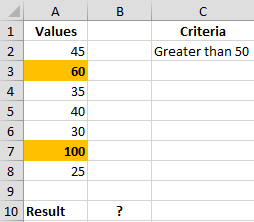In this case, we will use the COUNTIF function in VBA and using macro to view the result.
Following steps will guide you:

Click on Developer tab > Visual Basic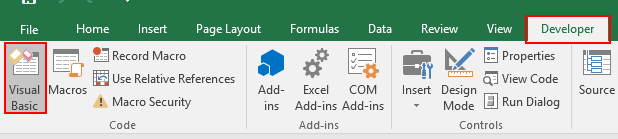Click on Insert > Module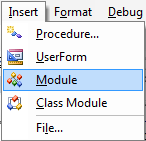This will create a module which can run on Excel
Enter the following code in the module

Sub Countif()Range(“B10?).Value = Application.WorksheetFunction.Countif(Range(“A2:A8?), “>” & 50)

End Sub

Explanation: This “Countif” module will counts the cells in range A2:A8 cells for the numbers which are greater than 50 and returns the total count in B10 cell

Now come to the worksheet and use the ALT + F8 shortcut key for opening Macro.

Under Macro window, select “Countif” module and click Run.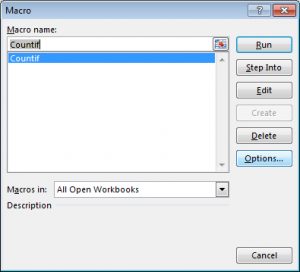Alternatively, you can press F5 to run the code in VBA screen.

After executing the macro we will get the output, refer to below snapshot.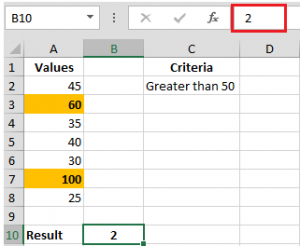NOTE: In above snapshot with VBA code you will only see the result, not the formula in cell B10.

VBA in Excel helps the user to build customized options and call whenever required. In this way, we can use COUNTIF in VBA code with one criteria. Hope you got this. You can perform this function in Excel 2016 and all the other versions. Find more articles on VBA and COUNTIF here. Mention any query down in the comment box below.

Popular Articles:

50 Excel Shortcuts to Increase Your Productivity

How to use the VLOOKUP Function in Excel

How to use the COUNTIF function in Excel

How to use the SUMIF Function in Excel

1.2.